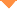首页 > 论文 > 激光与光电子学进展 > 56卷 > 11期(pp：110602--1)

Triangular Photonic Microwave-Signal Generation Based on Dual-Parallel Mach-Zehnder Modulator and Influence Factors of Its Similarity

• 摘要• 论文信息• 参考文献• 被引情况• PDF全文Abstract

The influence of the high-order Fourier components of an ideal triangular microwave signal on the similarity of the triangular signals is studied here. Using the numerical simulation method, the similarity between the original ideal waveform and the simulated waveform with different orders is studied. The numerical simulation results show that the similarity between the triangular photonic microwave signal and the ideal triangular microwave can reach 0.989 when the Fourier components of the triangular photonic microwave signal are retained to the third order. A scheme based on the generation of triangular microwave signals using a dual-parallel Mach-Zehnder modulator is presented. By controlling the six parameters of the system, a triangular photonic microwave signal can be obtained when the power ratio between the fundamental frequency and the third-order harmonics of the generated signal is equal to that between the first- and third-order Fourier components of the ideal triangular waveform. The experimental results show that the system can generate triangular photonic microwave signals with a repetition rate of 6 GHz.Yayuan Sun, Guangfu Bai, Lin Hu. Triangular Photonic Microwave-Signal Generation Based on Dual-Parallel Mach-Zehnder Modulator and Influence Factors of Its Similarity[J]. Laser & Optoelectronics Progress, 2019, 56(11): 110602